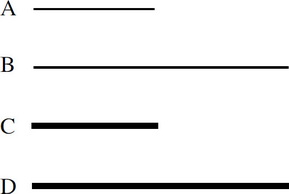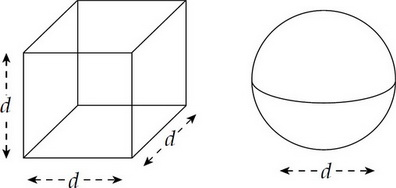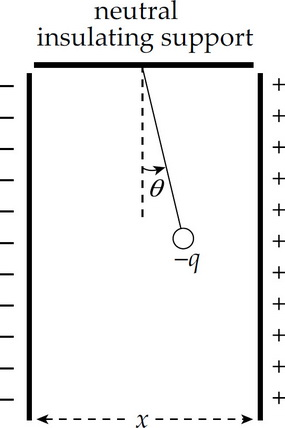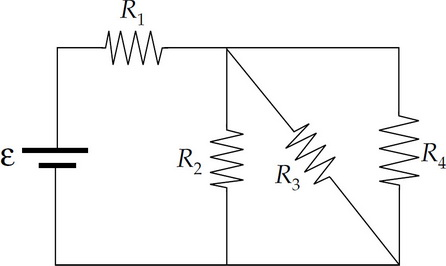# AP Physics C: Electricity and Magnetism Practice Test 1

### Test Information10 questions13 minutes

1. A nonconducting sphere is given a nonzero net electric charge, +Q, and then brought close to a neutral conducting sphere of the same radius. Which of the following will be true?

2. Which of the following would increase the capacitance of a parallel-plate capacitor?

3. Each of the following ionized isotopes is projected with the same speed into a uniform magnetic field B such that the isotope's initial velocity is perpendicular to B. Which combination of mass and charge would result in a circular path with the largest radius?

4. An ellipsoid-shaped conductor is negatively charged. Which one of the following diagrams best illustrates the charge distribution and electric field lines?

5.The four wires shown above are each made of aluminum. Which wire will have the greatest resistance?

6. Which of the following is NOT equal to one tesla?

7.The figure above shows two Gaussian surfaces: a cube with side length d and a sphere with diameter d. The net electric charge enclosed within each surface is the same, +Q. If ΦC denotes the total electric flux through the cubical surface, and ΦS denotes the total electric flux through the spherical surface, then which of the following is true?

8.The figure above shows two large vertical conducting plates that carry equal but opposite charges. A ball of mass m and charge –q is suspended from a light string in the region between the plates. If the voltage between the plates is V, which of the following gives the angle θ?

9. An object carries a charge of –1 C. How many excess electrons does it contain?

10. Each of the resistors shown in the circuit below has a resistance of 200 Ω. The emf of the ideal battery is 24 V.How much current is provided by the source?